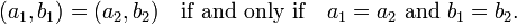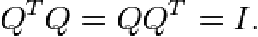Pathways To Learning... Since 2005 Hong Kong Registered School 566985

# O - oblate to overdetermined system of equations - Mathematics Dictionary

A   B   C   D   E   F   G   H   I   J   K   L   M   N   O   P   Q   R   S   T   U   V   W   X   Y   Z

oblate: A type of ellipsoid formed by squashing a sphere along one direction, or alternatively, stretching the sphere along two perpendicular directions.

oblique angle: An angle which is not a multiple of right angles (90°).

oblique coordinate system: Any coordinate systems where the coordinate axes are not perpendicular to each other.

oblique pyramid: A pyramid where the apex (the vertex not on the base) is not directly above the centreof the base.

oblique triangle: A non right-angled triangle.

oblong: A rectangle which is not a square.

obtuse angle: An angle greater than a right angle (90°) and less than 2 right angles (180°).

obtuse triangle: A triangle that has one (interior) angle greater than a right angle (90°).

octagon: A 8-sided polygon..

octahedron: A polyhedron which has eight faces. e.g. the dual of a cube.

octal system: A number system with base 8. (As opposed to the decimal system which has base 10.)

octant: One of the eight regions in a Cartesian coordinate system in 3-dimensions separates by planes which contain 2 axes each.

odd function: A function where the additive inverse of the value of the function with any argument is the value of the function with the additive inverse of the original as argument. i.e. f(-x) = -f(x) for all x

The graph of an odd function is rotationally symmetric about the origin.

odd number: A positive integer not divisible by 2. Alternatively, any positive integers which are not even numbers..

odds: The probability of an event expressed as a ratio of the probability of an event to the probability of the complement event (i.e. the event not happening).

one dimensional: The property of a line where each point has only 2 directions possible, usually indicated as positive and negative without loss of generality.

one-to-many correspondence: A mapping where one argument corresponds to many values. Such a mapping is not a function by definition.

one-to-one correspondence: The relation between 2 sets where there is a one-to-one function between them.

one-to-one function: A function which takes its domain in one set and codomain as the other, where the inverse function also exists. So that any element from either set CAN correspond to an element from the other set. Also known as a bijection.

open curve: A curve which is not closed, i.e. a curve where the endpoints do not coincide.

open region: A set of points on Rn where none of the points are on the perimeter of the set.

operand: The arguments (inputs) of an operator.

operator: The symbol denoting the application of an operation on operands by being placed beside them. For unary operations, the operator usually precedes the operand; while for binary operators, the operator is usually placed between the operands.

Note that for some operations, operators aren't often used (e.g. multiplication, exponentiation etc.) and in some cases an operator may be even exist for an operation. (For uncommon operations, they would have to be explicitly defined in each work.)

opposite: 1. A point on the intersection of the perimeter and a line through a specified point and the centre.

2. An edge neither of whose endpoints are the specified vertex in a triangle.

3. A vertex which is not an endpoint of an edge of a triangle.

4. A side parallel to the specified side in a regular polygon.

5. The geometric figure of the same type as the one specified, equidistant from the line of reflection, in a reflectively symmetric shape.

oppositely congruent: Two geometric figurescongruent with the opposite orientation - i.e. through a combination of translations, rotations and exactly one reflection.

orbit: The closed curve trajectory followed by a object under the influence of a central force.

order: 1. Polynomial - the integer exponent which is the greatest amongst all the terms in a polyomial

2. Derivatives - the number of iterated differentiation of an expression

2.Differential Equation - the order (in the sense of 2) of the highest-order derivative in a differential equation.

3. Curve - The greatest sum of the exponents of all variables within a single term of the equation representing the curve.

4. Matrix - the dimensions of a matrix

5. Rotational symmetry - the number of times a shape resemble itself in a revolution (where the beginning and the end of a revolution counts as only one).

ordered pair: A set of 2 elements where the order of the elements matter. i.e. 2 sets with the same 2 elements, but in reverse orders are considered different ordered pairs.

A common construction for the ordered pair (a,b) = { a , {a,b} }ordered set: A 2 or more member analogue of an ordered pair. Also known as a k-tuple.

ordinal number: A number denoting position (ordinal - nth), as opposed to magnitude (cardinal - size n), in a sequence.

ordinary differential equation: ODE - A differential equation without any partial derivatives.

origin: The central reference point of a coordinate system.

orthocenter: The intersection of the three line segments through the three vertices and perpendicular to the correpsonding opposite side in a triangle.

orthogonal: The property of a set of mathematical objects which are (pair-wise) perpendicular to each other..

orthogonal matrix: A matrix whose inverse is its transpose..orthogonal transformation: A transformation represented by an orthogonal matrix.

orthogonal vectors: A set of vectors which are all (pairwise) perpendicular to each other..

orthonormal: The property of a mathematical object which is in some sense orthogonal and normalized. e.g. matrix - the rows, considered as vectors, are all unit vectors perpendicular to each other; the same with the columns.

oscillating product: A bounded divergent product or a product which tends towards both positive and negative infinity.

oscillating sequence: A bounded divergent sequence or a sequence which tends towards both positive and negative infinity.

oscillating series: A bounded divergent series or a series which tends towards both positive and negative infinity.

oscillation: A periodic behavious.

outcome: The result of performing experiments.

outer product: The outer product of two vectors (x1, x2, x3, ... , xm) and (y1, y2, y3, ... , yn) is the matrix with elements mi,j = xi.yj

Alternatively, the outer product of vectors x and y is the matrix xTy

outlier: A data point judged to be not under consideration or not fitting of a general pattern within a data set.

oval: Any shape resembling elongated circles.

overdetermined system of equations: A system of equations with no solutions where all equations (by themselves) admit at least one solution set(often more).

A   B   C   D   E   F   G   H   I   J   K   L   M   N   O   P   Q   R   S   T   U   V   W   X   Y   Z

Genius is one percent inspiration and ninety-nine percent perspiration.